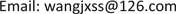﻿ 咸阳市城市建设用地利用绩效评价研究 Study on Performance Evaluation of Urban Construction Land Use in Xianyang

Urbanization and Land Use
Vol.05 No.04(2017), Article ID:22787,7 pages
10.12677/ULU.2017.54005

Study on Performance Evaluation of Urban Construction Land Use in Xianyang

Jianxing Wang, Shifang Liao

School of Resources and Environment & Historical Culture, Xianyang Normal University, Xianyang ShaanxiReceived: Nov. 4th, 2017; accepted: Nov. 17th, 2017; published: Nov. 24th, 2017ABSTRACT

In order to comprehensively evaluate the utilization of construction land in Xianyang, 14 evaluation indexes are constructed from four aspects. The comprehensive index method and barrier model are used to calculate the performance index and barrier of land use. The results showed that the performance of construction land use in Xianyang continued to improve from 2007 to 2014, and the comprehensive performance index increased from 0.0331 in 2007 to 0.9645 in 2014. The most important factor restricting construction land performance is land investment and land use efficiency.

Keywords:Construction Land Use, Performance, Obstacle Degree, Xianyang City1. 引言

2. 研究区域概况

3. 研究方法和数据来源

3.1. 研究方法

3.1.1. 评价指标体系构建Table 1. Evaluation index system of construction land performance in Xianyang

3.1.2. 指标标准化

${r}_{ij}=\frac{{x}_{ij}-\mathrm{min}{x}_{ij}}{\mathrm{max}{x}_{ij}-\mathrm{min}{x}_{ij}}$ ; (1)

${r}_{ij}=\frac{\mathrm{max}{x}_{ij}-{x}_{ij}}{\mathrm{max}{x}_{ij}-\mathrm{min}{x}_{ij}}$ ; (2)

3.1.3. 确定指标权重

① 计算每个指标值的比重：

${M}_{ij}={r}_{ij}/{\sum }_{1}^{8}{r}_{ij}\text{\hspace{0.17em}}\text{\hspace{0.17em}}\text{\hspace{0.17em}}\left(i=1,2,\cdots ,8;j=1,2,\cdots ,14\right)$ (3)

② 计算第q项指标的熵值：

${W}_{q}=-{\sum }_{1}^{8}{M}_{ij}\mathrm{ln}{M}_{ij}/\mathrm{ln}8$ (4)

③ 计算指标的差异系数值：

${g}_{q}=1-{W}_{q}$ (5)

④ 计算指标的权重值：

${k}_{q}={g}_{q}/{\sum }_{1}^{14}{g}_{q}$ (6)

3.1.4. 土地利用绩效评价模型

${Z}_{b}={\sum }_{1}^{4}{\sum }_{1}^{14}{k}_{q}×{r}_{ij}$ (7)

3.1.5. 障碍度模型

${F}_{q}={C}_{q}×{W}_{q},\text{\hspace{0.17em}}\text{\hspace{0.17em}}\text{\hspace{0.17em}}{H}_{q}=1-{S}_{q}$ (8)

${V}_{q}=\frac{{H}_{q}×{F}_{q}}{{\sum }_{1}^{14}\left({H}_{q}×{F}_{q}\right)}×100%$ (9)

3.2. 数据来源

4. 结果与分析

4.1. 2007~2014年咸阳市建设用地利用绩效分析

“土地利用程度、土地利用效率、土地利用生态可持续性、土地投入水”四个准则层的土地利用绩Table 2. Performance index of construction land in Xianyang from 2007 to 2014

4.2. 影响建设用地利用的障碍因子分析Table 3. Main index’s obstacle of construction land in xianyang Unit: %

5. 结论与建议

5.1. 结论

5.2. 建议

1) 坚持走城市生态可持续发展之路

2) 优化建设用地结构，提高土地利用绩效

Study on Performance Evaluation of Urban Construction Land Use in Xianyang[J]. 城镇化与集约用地, 2017, 05(04): 31-37. http://dx.doi.org/10.12677/ULU.2017.54005

1. 1. 班茂盛, 方创琳. 北京高新技术产业区土地利用绩效综合评价[J]. 地理学报, 2008, 64(3): 154-162.

2. 2. 田光进, 刘纪远, 庄大方, 等. 基于遥感GIS的20世纪90年代中国城镇用地时空特征[J]. 第四纪研究, 2003, 23(4): 421-427.

3. 3. 咸阳市统计局. 咸阳统计年鉴: 2015 [M]. 北京: 中国统计出版社, 2016.

4. 4. 周丹丹. 城市土地利用绩效评价研究[D]: [硕士学位论文]. 重庆: 重庆大学, 2010.

5. 5. 谭术魁, 郑君怡, 李雅楠, 等. 湖北省城市土地利用绩效评价及障碍度分析——基于改进TOPSIS法[J]. 中国房地产, 2015, 2(12): 37-46.

6. 6. 陈士银, 周飞, 吴雪彪. 基于绩效模型的区域土地利用可持续性评价[J]. 农业工程学报, 2009, 25(6): 249-253.